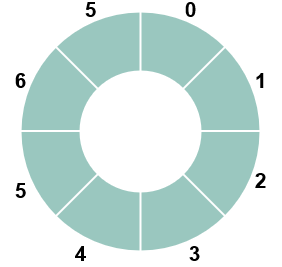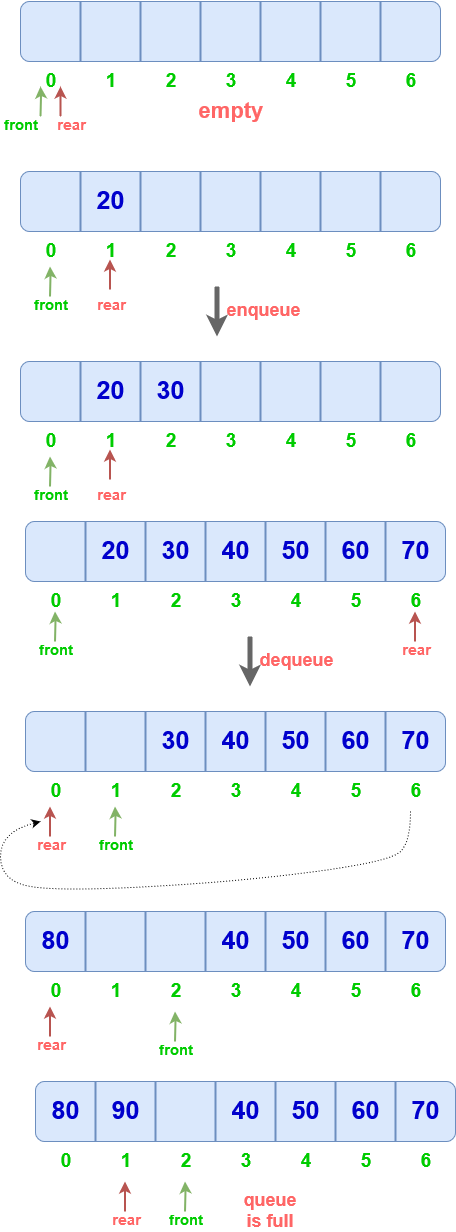# Circular Queue Data Structure

## Circular Queue

Circular queue is a modified form of queue in which the front and rear pointers move circularly.
Instead of starting from -1, now front and rear start from 0.

Representation of circular queue:``````class Queue{
int arr
int front, rear;
int size;
};

Queue(int n)
{
front = 0;
rear  = 0;
size =n;
}
``````

## Operations

To make front and rear move circularly, after rear reaches (size-1 ) position, it must be reset to 0.
To do this, '%' is used.
Working of mod operator can be seen:
Let size = 5.
| Rear = | (Rear+1)%size |
|:–:|:–:|
| 0 | (0+1)%7 = 1 |
| 1 | (1+1)%7 =2|
| 2 | (2+1)%7 =3|
| : | : |
| 4 | (4+1)%5 =0 |

Hence when rear points to (size-1), it gets reset to 0.

## Working of circular queue :### Enqueue

• Elements are inserted from the rear end as usual.
• If rear reaches (size-1), it is reset to zero
• Insertion can't be done at the index where front is pointing

Only (size-1) spaces can be utilized in Circular queue.

``````void enqueue(int x)
{
if((rear+1)%size == front)
print("Queue is Full");
else
{
rear=(rear+1)%size;
arr[rear]=x;
}
}
``````

### Dequeue

``````int dequeue()
{
int x=-1;
if(front==rear)
print("Queue is Empty")
else
{
front=(front+1)%size;
x=arr[front];
}
return x;
}
``````

### Display

``````void Display()
{    int i= front+1;
do
{
print(arr[i]);
i=(i+1)%size;
}while(i!=(rear+1)%size);
}
``````

Complexity for all operations on circular queue is O(1).

Scroll to Top

### Full Stack Integrated Bootcamp Free Trial

• Please enter a number from 7000000000 to 9999999999.